## Monday, August 19, 2013

### Fast (and pretty easy) Currency Conversion without Calculators

With the prevalence of mobile phones, it's easy to convert between currencies by simply using the phone's calculator. If you're like most people, however, you won't be doing this while shopping on the street markets of Thailand or anywhere else abroad, provided the sum is not too large (say anything below 100 USD).

Here's a practical way to convert between currencies fast and easy, all done in your head.

Consider some practical examples.

USD to EUR conversion
We want to convert from USD to Euros (EUR).
Google tells me that today, 1 USD = 0.75 EUR.
How much is 67 USD in EUR?

Note that 0.75 = 3/4, so we have 67 * 3 / 4. From a mathematical point of view, it doesn't matter if we multiply 67 by 3 first, and then divide it by 4, or if we divide 67 by 4 first, then multiply by 3. It is however much easier from humans (and computers!) to deal with small numbers, so we start by dividing to get a smaller number.

How do you divide 67 by 4? The answer is that you half it two times: 67 / 4 = 67 / (2*2) = 67 / 2 / 2.
How do you halve 67? 67 is 60+7, and if we take half of each and then add them, we'll have the correct answer. Half of 60 is 30, and half of 7 is approximately 4 (we stick to integers). Thus the answer is 30+4 = 34. Repeating once more (remember, we halve two times), 34 = 30+4, and halving each gives 15+2 = 17.

Now, we multiply 17 by 3 using the same technique of splitting 17 into 10+7 and then multiplying (instead of dividing) by 3: 3*10 = 30, and 3*7 = 21, so you get 30+21 = 51.

In one go: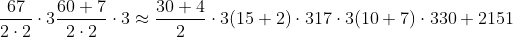67 USD is approximately 51 EUR. The exact answer is 50.25 EUR.

What you need to remember is thus:
1. The general operations: halve the number two times, then multiply by 3
2. How to halve and multiply by 3 using the "splitting" trick.
Let's we want to do the opposite now: convert euros into USD. 1/0.75 = 1.33, so 1 EUR = 1.33 USD.

Let's say we have 247 EUR. This is 247*1.33 USD, but how do we calculate it easily?

First, we omit the second decimal, i.e. 1.33 is approximately 1.3. So we only need to do 247 * 1.3. First, using the splitting trick, we see that: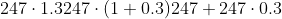So the hard part is a little easier now: we need to estimate 247*0.3. Noting that 0.3 = 3/10, and doing the division before multiplication as before, we get: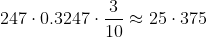So the answer is 247 + 75. A rough estimate is given by 250+75 = 250 + 50 + 25 = 325.

So 247 EUR is approximately 325 USD. The exact answer is 328.51 USD.

It seems then that the trick is to come up with ways to multiply and divide by decimal numbers, such as 0.75 and 0.3. This can always be done, since all conversion rates are rational numbers, meaning they can be written in the form Integer/Integer. By using a fairly simple numerator and denumerator, we can then perform the estimation fast. Multiplication is in general easy, division much harder. Here's a couple of tricks:

Divide by 2 (halving)
This is simply taking half the quantity. If you need to divide say 573 by 2, then use the splitting technique: 573 = 500 + 70 + 3. Halving each gives approximately 250 + 35 + 2 = 250 + 37 = 287.

Divide by 4 and 8 (halving many times)
Also very easy: dividing by 4 is the same as halving twice. Dividing by 8 is the same as halving three times.

Divide by 10
The easiest to do. 128 divided by 10 is 12.8. In general, XYZ divided by 10 will be XY.Z, where X,Y and Z are digits (0,1,2,3,...,9). We then simply round to nearest integer> 12.8 is closest to 13.

Divide by 5 ("divide by 10 and double")
This one's really easy too: x/5 = x/10*2, so all you need to do is divide by 10 (using the method above), and then double the result. Example: 128/5 can be calculated first dividing 128 by 10, giving 13. Doubling gives 26.

Divide by 3 ("take average of 2 and 5")
This one's a bit harder. A nice way to estimate x/3 that builds on the techniques already mentioned is to take the average of x/2 and x/5.
Example: 175 divided by 3 can be approximated by noting that:
175/5 = 18*2 = 36
175/2 = (100 + 75 + 5) / 2 = 50 + 37 + 3 = 50 + 40 = 90
Taking averages, we get (36+90)/2 = 126/2 = (100+20+6)/2 = 50 + 10 + 3 = 63.
In general, this technique gives (x/2 + x/5)/2 = 7x/20 = 0.35x, instead of 0.3333x.
(It may seem more intuitive to take average of x/2 and x/4, but this gives a worst estimate of 0.375x.)

Divide by 7 ("take average of 5 and 10")
Also a bit harder. When doing x/7, we can take the average of dividing by 5 and 10.
384/7 is somewhere between:
384/10 = 38
384/5 = 38*2 = (30+8)*2 = 60 + 16 = 76
Taking averages, we get (38+76)/2 = (100 + 14)/2 = 50 + 7 = 57
The exact answer is close to 55.
This techniques calculates (x/5 + x/10)/2 = 3x/20 = 0.15x instead of x/7 = 0.143, so it's a good estimate for relatively small sums of money.

Here's another example. 1 THB (Thai Baht) is 0.032 USD. How much is the saleswoman asking for in USD when she says 650 THB? The answer is 650*0.032 USD. To estimate this quantity, we manipulate the conversion rate 0.032 into rules of the form above. Note that 0.032 = 32/1000 which we approximate as 30/1000 = 3/100 (32/1000 is (30+2)/1000 = 3/100 + 2/1000, so we're skipping a term containing two parts in 1000).

Calculating 650 * 3/100 is easy: do the division first (dividing by 100 is dividing by 10 two times, since 100 = 10*10). We then get 6.5, which we round to 7. Multiplying by 3 gives 21. Here's the full estimate in one line: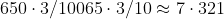Let's now convert from GBP (Brittish pound) to USD. 1 GBP = 1.56 USD. How much is Harrods asking you in USD when they say 489 GBP?

First we deal with the conversion rate. 1.56. This is close to 1.6, which we instead use. 1.6 = 16/10, and looking at prime factors we have: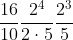This works: we can divide by 5 and then double 3 times. It's however rather tedious, as if we allow a little more rounding error, 1.56 is also close to 1.5, which gives: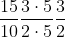A much simpler calculation: we half, then multiply by 3.
Thus, estimating 489 GBP is done as follows:
489/2 = (400 + 80 + 9)/2 = 200 + 40 + 5 = 245.
245 * 3 = (200 + 40 + 5)*3 = 600 + 120 + 15 = 720 + 15 = 735.

489 GBP is approximately 735 USD (although for such large amounts I would suggest you use a calculator!).